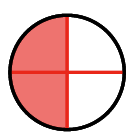### Home > ACC6 > Chapter 6 Unit 6 > Lesson CC1: 6.1.1 > Problem6-12

6-12.

For each of the following questions, draw a diagram to explain your answer.

1. How many fourths are in one half?2. How many sixths are in two thirds?

Try using a number line for your diagram.
First divide it into thirds, then sixths.

3. How many fourths are in six eighths?

Simplifying the fraction will give you the answer.

4. How many halves are in $3\frac{5}{10}$?

Try making this one a fraction greater than one instead of a mixed number.